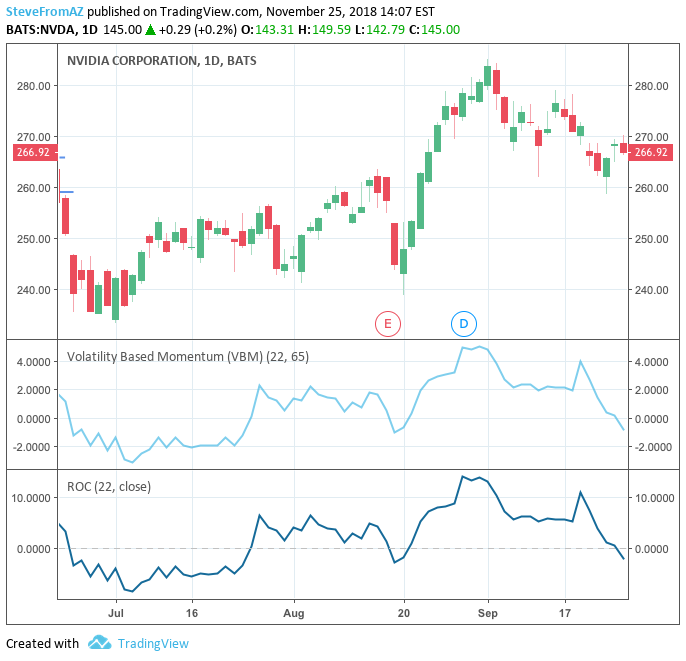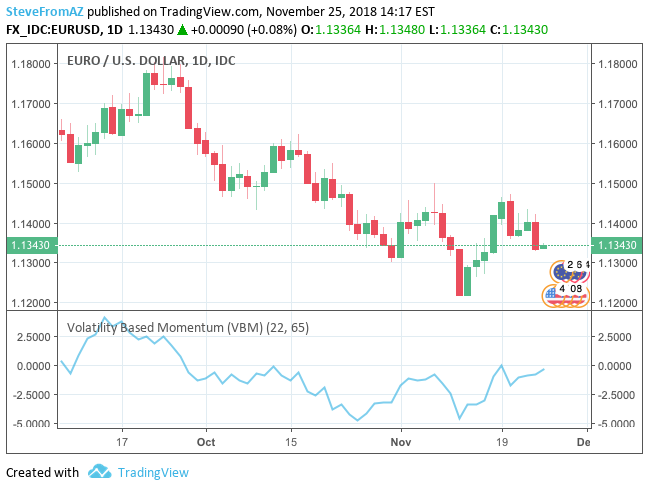# A Volatility Based Momentum Indicator for Traders

### Steve Roehling

November 25, 2018

Fundamentally, trading is about analyzing the supply and demand of a security (asset which can be traded), such as stocks, commodities, or Forex pairs. A trader then makes decisions to purchase or sell these securities, ideally for a profit. When entering a trade, there are numerous factors to take into consideration, such key price levels, liquidity, and momentum.

Momentum is the speed of movement for a security’s price. With respect to supply and demand, a security with strong momentum is experiencing an imbalance of demand over supply when price is rising, or supply over demand when price is falling. To the extent momentum is directly and fundamentally related to supply and demand, it is very important for traders to have a means to measure and analyze a security’s momentum.

The most pure and simple calculation of momentum is the following:

`(Closing price today)- (Closing price n periods ago)`

Going beyond a simple momentum calculation, there are numerous technical analysis indicators a trader can use for a more sophisticated analysis of momentum. For example, the rate of change (ROC) indicator measures the percent change in price from one period to the next. However, since many traders orient their trading around volatility, a variation on ROC which is based upon volatility rather than percentages offers numerous advantages.

### Starting with the Rate of Change Indicator

The calculation of the percentage-based ROC indicator is the following:

`ROC(n) = [(Close — Close n periods ago) / (Close n periods ago)] * 100`

The ROC indicator shows when prices are surging or plunging. There is no upper bound to the values from this indicator, but the maximum a price could plunge is 100%.

However, a primary shortcoming of the ROC indicator is its use of percentages as a unit. For example, using percentages, one cannot compare the momentum of two different stocks side by side, without first knowing what is a normal percentage gain or loss is for each stock.

### Calculating Volatility Based Momentum

Instead of basing the rate of change on percentages, expressing momentum in ‘multiples of volatility’ (MoV) is another alternative. The calculation for a volatility based momentum (VBM) indicator is very similar to ROC, but divides by the security’s historical volatility instead. The average true range indicator (ATR) is used to compute historical volatility.

`VBM(n,v) = (Close — Close n periods ago) / ATR(v periods)`

For example, on a daily chart, VBM(22,65) calculates how many MoV price has increased or decreased over the last 22 trading days (approximately one calendar month).

Besides having a different unit than ROC, the VBM indicator has almost an identical shape as the ROC indicator. Therefore, any technical analysis and interpretation which would apply to ROC, applies equally to VBM. Along these lines, below is an example chart for NVDA; this chart illustrates the similar shapes of the ROC and VBM indicators, even though they have different units:The chart used in the example above was created using the TradingView charting website. In TradingView, custom indicators can be created using their scripting language. The VBM indicator script used in this example has been published on TradingView.

### Trading Based On Multiples of Volatility (MoV)

The key difference between VBM and ROC indicators is the VBM indicator uses multiples of volatility (MoV) as its unit of measurement, instead of a percentage gain or loss.

For traders who factor volatility more prominently than percentages into their trading, VBM is arguably a more suitable alternative than ROC. Traders can incorporate volatility in numerous ways. For example, using the ATR indicator as a measure of volatility, traders can use volatility in their assessment of risk versus reward, calculating stop losses, determining price targets, and calculating position sizes. By using VBM as a momentum indicator, traders can also evaluate momentum in terms of volatility.

### Benefits of a Volatility Based Momentum Indicator

The VBM indicator is a very simple variation on ROC. Given the importance of volatility to many traders, I’d be surprised if other traders aren’t already using something very similar, if not the same  . Whether or not VBM is an original concept, by elaborating on the numerous benefits of VBM vs ROC, hopefully other traders can benefit from the use of VBM as a technical analysis indicator.

Using VBM, MoV effectively serves as a normalized, universal unit of measurement for momentum. By simply using MoV instead of percentages as a unit, the VBM indicator realizes numerous advantages.

First, the VBM indicator can be used with any type of security, such as stocks or Forex. Unlike ROC, there is no need to reinterpret what a percent gain or loss means for each. For example, below is a chart of the EUR/USD Forex currency pair; using the VBM indicator, the values can be interpreted in a similar manner as stocks/equities:Using VBM, different stocks can be compared side by side without having to discern what a normal percentage gain or loss is for the stock in question. For example, traders will often have a watch list with numerous stocks under consideration. Using charting software, a trader will periodically cycle through a list of charts for each stock, re-evaluating each one for possible entry. Using the VBM indicator, a trader can readily interpret the momentum of individual stocks, or evaluate a stock’s momentum in relation to other stocks in the watch list or the market as a whole.

Finally, VBM also works on multiple timeframes. Traders will often evaluate an individual security on multiple timeframes, such and daily and weekly timeframes. Since VBM uses the same unit of measurement for all timeframes, momentum can be interpreted in a consistent manner.

### Summary and Next Steps

The VBM indicator offers numerous benefits to traders who orient their trading around volatility. For these traders, VBM expresses momentum in a normalized, universally applicable ‘multiples of volatility’ (MoV) unit. Given the universal applicability of MoV, VBM is especially suited to traders whose trading incorporates numerous timeframes, different types of securities(e.g., stocks, Forex pairs), or the frequent comparison of momentum between multiple instruments.

My own trading is definitely oriented around volatility. In this regard, going forward I’m interested to look at other technical analysis indicators to see if a variation with MoV as its unit is possible.

Footnotes:

 Even in my own trading, I’ve previously integrated volatility and momentum. When trading stocks on an intraday (day trading) basis, one useful measurement is a stock’s intraday price movement as a multiple of daily volatility. This measurement tells you if the intraday price movement is exceptional and has become “unhinged” from the daily price.

 Similar to ATR, the standard deviation of price or returns is another common measure of volatility. In the sharpe ratio, for example, a standard deviation is the denominator to normalize returns based upon risk and volatility. Also using a standard deviation, a “risk adjusted momentum” investment strategy has been previously studied. However, ATR is arguably better suited to traders who consistently use ATR to determine risk versus reward, stop losses and price targets.